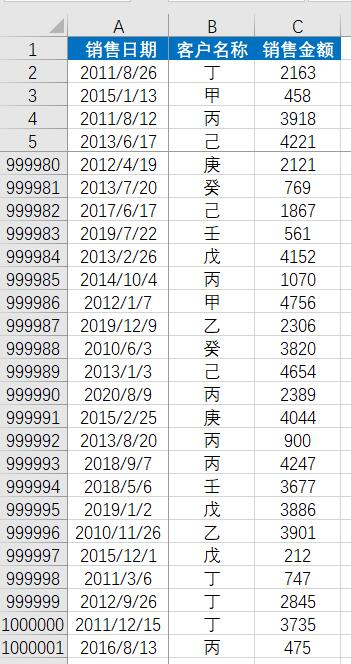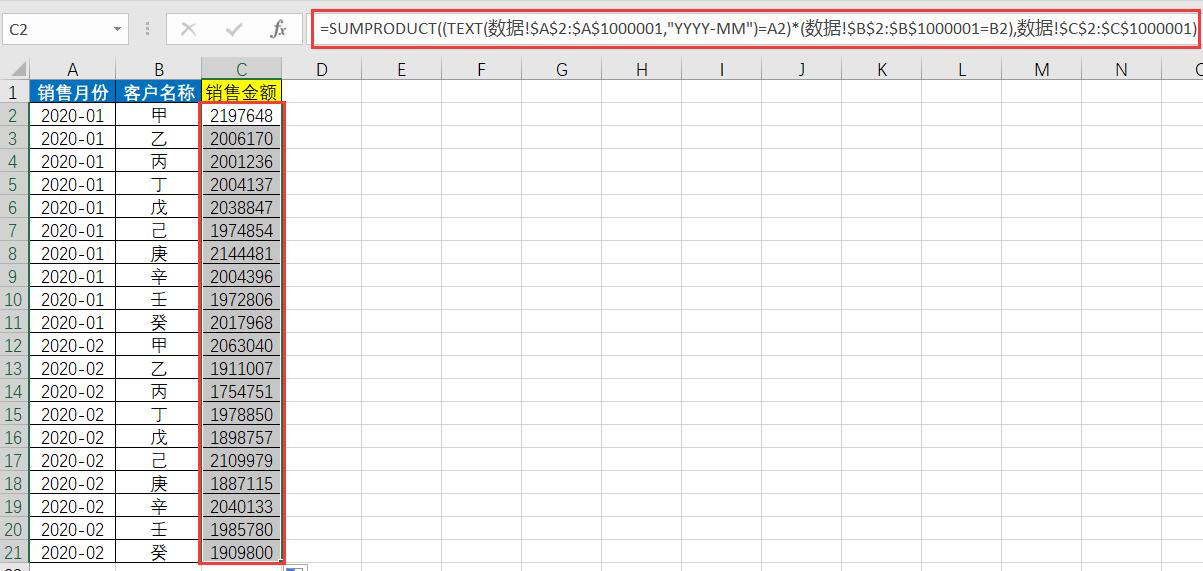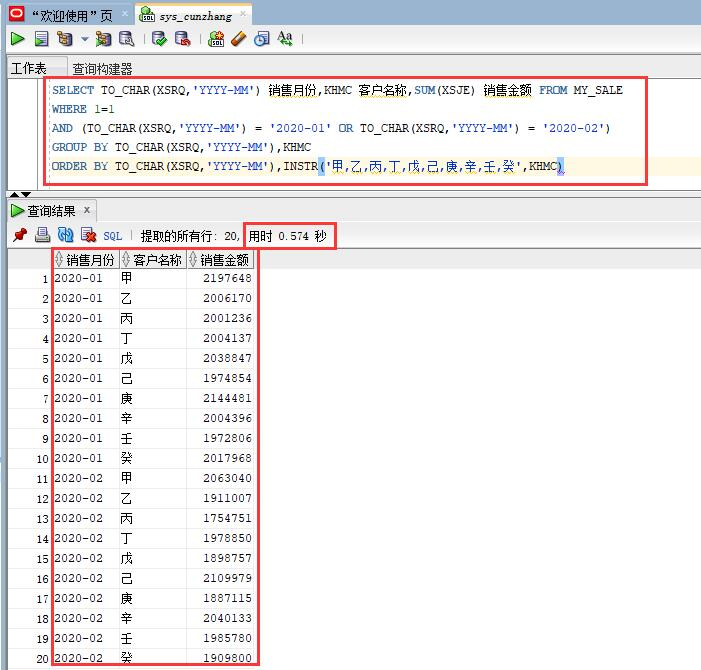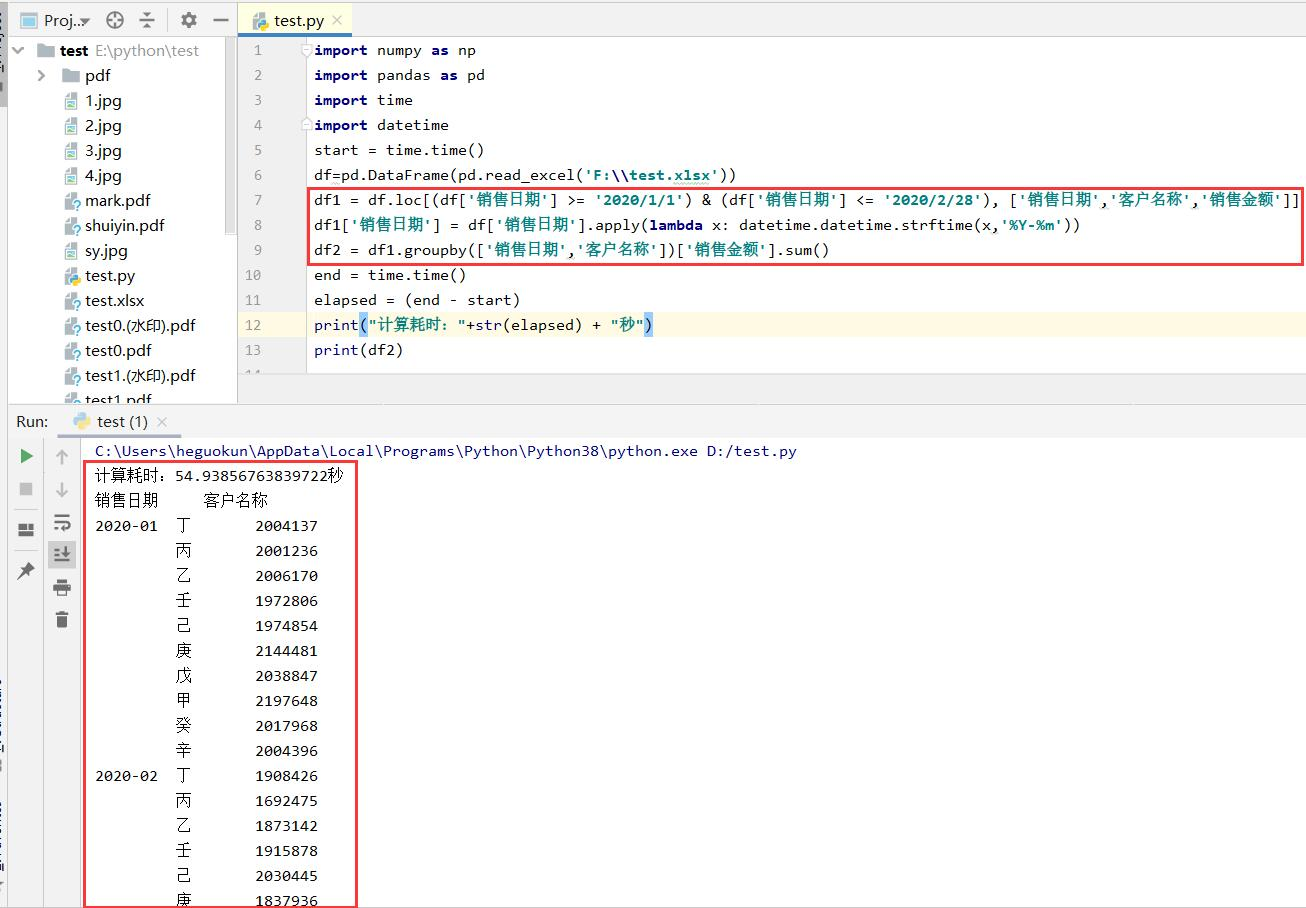# 百万数据在Excel、SQL与Python中的处理效率1、Excel处理=SUMPRODUCT((TEXT(数据!\$A\$2:\$A\$1000001,"YYYY-MM")=A2)*(数据!\$B\$2:\$B\$1000001=B2),数据!\$C\$2:\$C\$1000001)

2、SQL处理SQL语句：

SELECT TO_CHAR(XSRQ,'YYYY-MM') 销售月份,KHMC 客户名称,SUM(XSJE) 销售金额 FROM MY_SALE

WHERE 1=1

AND (TO_CHAR(XSRQ,'YYYY-MM') = '2020-01' OR TO_CHAR(XSRQ,'YYYY-MM') = '2020-02')

GROUP BY TO_CHAR(XSRQ,'YYYY-MM'),KHMC

ORDER BY TO_CHAR(XSRQ,'YYYY-MM'),INSTR('甲,乙,丙,丁,戊,己,庚,辛,壬,癸',KHMC)

SQL计算完成时间：0.574秒

3、Python处理Python代码：

```numpy np
pandas pd
time
datetime
start = time.time()
df1 = df.loc[(df[] >= ) & (df[] <= ), [,,]]
df1[] = df[].apply(x: datetime.datetime.strftime(x,))
df2 = df1.groupby([,])[].sum()
end = time.time()
elapsed = (end - start)
(+(elapsed) + )
(df2)```